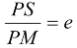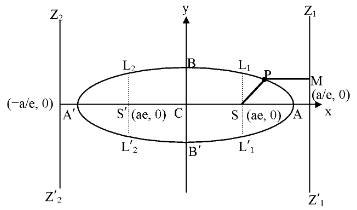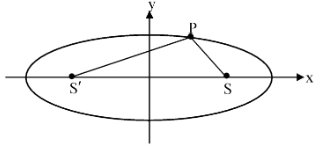# Introduction

## Ellipse of Class 11

### Definition

An ellipse is the locus of a point, which moves in such a way, that the ratio of its distances from a fixed point and from a fixed straight line is constant.

The fixed point is called focus, the fixed straight line is called directrix and the constant ratio is called eccentricity of the ellipse. Eccentricity is denoted by e and it is < 1,## Alternate Definition:

 An ellipse is the locus of a point which moves in such a way that the sum of its distances from two fixed points remains constant. PS + PS′ = 2a Remarks: The constant in above definition should be greater than the distance between the fixed points. This definition is also called the physicaldefinition of ellipse. With the help of this definition we can physicaly draw the ellipse by taking two clips at S and S′a loose thread of length 2a.